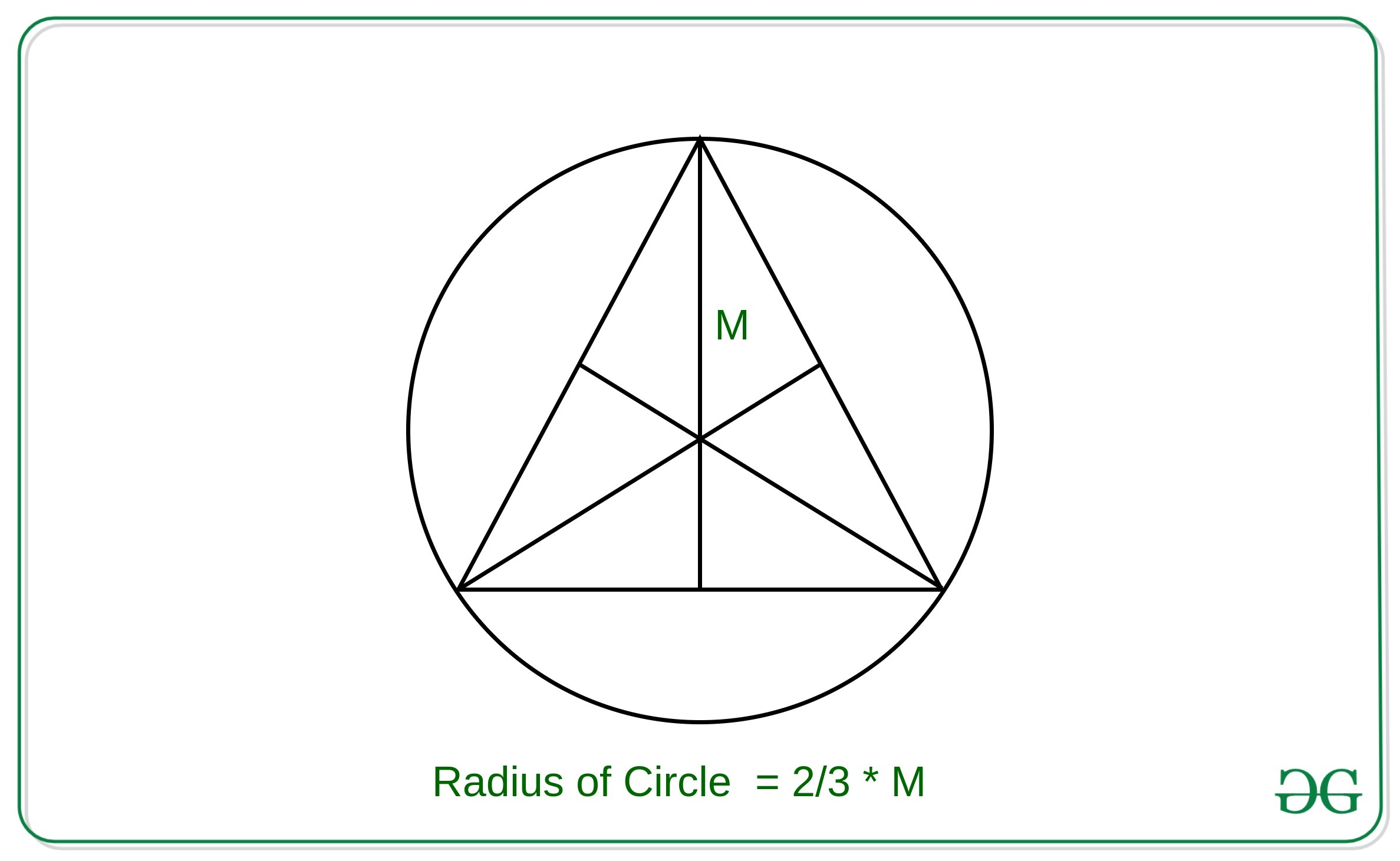# Area of Circumcircle of an Equilateral Triangle using Median

Given the median of the Equilateral triangle M, the task is to find the area of the circumcircle of this equilateral triangle using the median M.

Examples:

Input: M = 3
Output: 12.5664

Input: M = 6
Output: 50.2655

## Recommended: Please try your approach on {IDE} first, before moving on to the solution.

Approach: The key observation in the problem is that the centroid, circumcenter, orthocenter and incenter of an equilateral triangle all lie at the same point.Therefore, the radius of the circle with the given median of the equilateral triangle inscribed in the circle can be derived as:Then the area of the circle can be calculated using the approach used in this article

Below is the implementation of the above approach:

## C++

 // C++ implementation to find the  // equation of circle which  // inscribes equilateral triangle  // of median M     #include  const double pi = 3.14159265358979323846;  using namespace std;     // Function to find the equation  // of circle whose center is (x1, y1)  // and the radius of circle is r  void circleArea(double r)  {      cout << (pi * r * r);  }     // Function to find the  // equation of circle which  // inscribes equilateral triangle  // of median M  void findCircleAreaByMedian(double m)  {      double r = 2 * m / 3;         // Util Function to find the      // circle equation      circleArea(r);  }     // Driver code  int main()  {      double m = 3;         // Function Call      findCircleAreaByMedian(m);      return 0;  }

## Java

 // Java implementation to find the   // equation of circle which   // inscribes equilateral triangle   // of median M   import java.util.*;     class GFG{         // Function to find the equation   // of circle whose center is (x1, y1)   // and the radius of circle is r   static double circleArea(double r)  {      double pi = 3.14159265358979323846;      return (pi * r * r);  }         // Function to find the   // equation of circle which   // inscribes equilateral triangle   // of median M   static double findCircleAreaByMedian(int m)  {      double r = 2 * m / 3;             // Function call to find       // the circle equation      return circleArea(r);  }     // Driver code   public static void main(String args[])  {      int m = 3;             System.out.printf("%.4f", findCircleAreaByMedian(m));  }  }     // This code is contributed by virusbuddah_

## Python3

 # Python3 implementation to find the  # equation of circle which inscribes   # equilateral triangle of median M     pi = 3.14159265358979323846    # Function to find the equation  # of circle whose center is (x1, y1)  # and the radius of circle is r  def circleArea(r):             print(round(pi * r * r, 4))     # Function to find the  # equation of circle which  # inscribes equilateral triangle  # of median M  def findCircleAreaByMedian(m):             r = 2 * m /3        # Function to find the      # circle equation      circleArea(r)     # Driver code  if __name__ == '__main__':             m = 3        # Function call      findCircleAreaByMedian(m)     # This code is contributed by mohit kumar 29

## C#

 // C# implementation to find the   // equation of circle which   // inscribes equilateral triangle   // of median M   using System;     class GFG{          // Function to find the equation   // of circle whose center is (x1, y1)   // and the radius of circle is r   static double circleArea(double r)   {       double pi = 3.14159265358979323846;       return (pi * r * r);   }              // Function to find the   // equation of circle which   // inscribes equilateral triangle   // of median M   static double findCircleAreaByMedian(int m)   {       double r = 2 * m / 3;                  // Function call to find       // the circle equation       return circleArea(r);   }          // Driver code   public static void Main(string []args)   {       int m = 3;                  Console.WriteLine("{0:f4}", findCircleAreaByMedian(m));   }   }      // This code is contributed by AnkitRai01

Output:

12.5664


Attention reader! Don’t stop learning now. Get hold of all the important DSA concepts with the DSA Self Paced Course at a student-friendly price and become industry ready.

My Personal Notes arrow_drop_upCheck out this Author's contributed articles.

If you like GeeksforGeeks and would like to contribute, you can also write an article using contribute.geeksforgeeks.org or mail your article to contribute@geeksforgeeks.org. See your article appearing on the GeeksforGeeks main page and help other Geeks.

Please Improve this article if you find anything incorrect by clicking on the "Improve Article" button below.